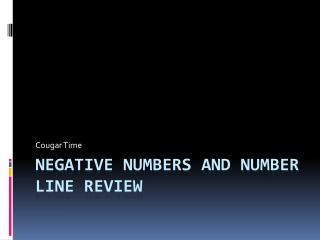DownloadDownload PresentationNegative numbers and number line review

# Negative numbers and number line review

Télécharger la présentation## Negative numbers and number line review

- - - - - - - - - - - - - - - - - - - - - - - - - - - E N D - - - - - - - - - - - - - - - - - - - - - - - - - - -
##### Presentation Transcript

1. Cougar Time Negative numbers and number line review

2. Adding Negative Numbers • What are the two rules for adding integers? • Same Signs = Add and keep the sign • Different Signs = Find the absolute value, subtract and keep the sign of the larger absolute value

3. Subtracting Negative Numbers • What is the rule for subtracting integers? • Same Change Flip • Same – Keep the first number the same • Change – Change subtraction to addition • Flip – Flip the sign of the second number

4. Practice • -12 + 6 • 13 + (-8) • (-16) + (-8) • -19 + 21 • -78 + -15 • 18 + -4 • -12 – (-15) • 34 – 78 • 23 – (-17) • 4 – 16 • -27 – 11 • -29 – (-8)

5. Multiplication and Division • What are the rules multiplying and dividing negative number? • Same Signs = Positive • Different Signs = Negative

6. Practice • 6 x (-8) • -5 x 20 • -8 x (-8) • 9 x (-11) • -14 x (-3) • 32 ÷ -8 • -120 ÷ -6 • -88 ÷ -11 • 54 ÷ -6 • -90 ÷ -15

7. Number Lines • Where on the number line are negative numbers? • LEFT of zero! • Where on the number line are positive numbers? • RIGHT of zero! (-) Negative 0 (+) Positive

8. Mixed Review • Draw a number line ranging from -25 to 25 • Write down the rules for adding and subtracting integers. • Write down the rules for multiplying and dividing integers.